• 多分类
万次阅读
2022-01-29 16:18:36

在针对一个网站的RPA技术中，验证码识别也是一个很常见的需要解决的问题。如下图所示的验证码：前面在我的博客中所讲的内容是单标签多分类，例如一个手写数字图片，分出10类，得到这个图片表示的1个字符信息，而验证码一般是多个字符，每一个字符均有多个类别，因此验证码识别的问题就属于多标签多分类问题，在这里不能用之前识别手写数字的方法来进行验证码识别。
本文的开发环境如下：
tensorflow 2.5.0
本文所用的训练样本如下：
下载链接

# 1 多标签多分类之验证码识别模型的训练

多标签多分类的处理要点是验证码的几个字符，每个字符均变换成独热编码，例如本文所示的验证码字符种类为36个（10个数字以及26个大写英文字母），验证码包含了4个字符，处理流程先把每一个字符变成one-hot，最后把4个字符的one-hot编码拼接成一行。因此每个字符的标签长度为36x4=144个。
例如对于验证码的4个字符为：LKXE
字符集为：0123456789ABCDEFGHIJKLMNOPQRSTUVWXYZ
计算4个字符中的每个字符对应字符集的下标为：
[21, 20, 33, 14]
4个字符的one-hot编码为：

[[0. 0. 0. 0. 0. 0. 0. 0. 0. 0. 0. 0. 0. 0. 0. 0. 0. 0. 0. 0. 0. 1. 0. 0.
0. 0. 0. 0. 0. 0. 0. 0. 0. 0. 0. 0.]
[0. 0. 0. 0. 0. 0. 0. 0. 0. 0. 0. 0. 0. 0. 0. 0. 0. 0. 0. 0. 1. 0. 0. 0.
0. 0. 0. 0. 0. 0. 0. 0. 0. 0. 0. 0.]
[0. 0. 0. 0. 0. 0. 0. 0. 0. 0. 0. 0. 0. 0. 0. 0. 0. 0. 0. 0. 0. 0. 0. 0.
0. 0. 0. 0. 0. 0. 0. 0. 0. 1. 0. 0.]
[0. 0. 0. 0. 0. 0. 0. 0. 0. 0. 0. 0. 0. 0. 1. 0. 0. 0. 0. 0. 0. 0. 0. 0.
0. 0. 0. 0. 0. 0. 0. 0. 0. 0. 0. 0.]]


将4个字符的one-hot编码拼接成一行：

[0. 0. 0. 0. 0. 0. 0. 0. 0. 0. 0. 0. 0. 0. 0. 0. 0. 0. 0. 0. 0. 1. 0. 0.
- 0. 0. 0. 0. 0. 0. 0. 0. 0. 0. 0. 0. 0. 0. 0. 0. 0. 0. 0. 0. 0. 0. 0.
- 0. 0. 0. 0. 0. 0. 0. 1. 0. 0. 0. 0. 0. 0. 0. 0. 0. 0. 0. 0. 0. 0. 0.
- 0. 0. 0. 0. 0. 0. 0. 0. 0. 0. 0. 0. 0. 0. 0. 0. 0. 0. 0. 0. 0. 0. 0.
- 0. 0. 0. 0. 0. 0. 0. 0. 1. 0. 0. 0. 0. 0. 0. 0. 0. 0. 0. 0. 0. 0. 0.
- 0. 1. 0. 0. 0. 0. 0. 0. 0. 0. 0. 0. 0. 0. 0. 0. 0. 0. 0. 0. 0. 0. 0.]


以上就是多标签多分类的标签数据处理流程。
对于多标签多分类的网络结构有如下几个要点：

• 隐藏层激活函数用relu
• 输出层的输出个数为每个标签的总数(拼接成一行的one-hot编码个数）,激活函数为sigmoid
• 损失函数采用二元交叉熵binary_crossentropy
• 监控指标采用自定义回调函数计算准确率

训练的代码如下：

'''
利用多标签多分类实现验证码识别
作者：还魂草
'''
from matplotlib.pyplot import axis
import numpy as np
import string
import os
from PIL import Image
import random
from tensorflow.keras.preprocessing import image as kimage
from tensorflow.keras import models
from tensorflow.keras import layers
from tensorflow.keras import callbacks

#验证码的所有字符组合，本次识别的验证码只包含数字以及小写英文字母
characters=string.digits+string.ascii_uppercase
#验证码字符长度
yzm_len=4

model=None

#训练样本和测试样本的目录
yzm_train_dir='./yzm/train/'
yzm_test_dir='./yzm/test/'
#获取目录中的验证码路径和验证码的名称
def get_allImgInfo(img_dir):
yzmInfo=list()
for filename in os.listdir(img_dir):
yzm_temp=list()

#验证码的名称
yzm_name=filename[0:-4]
#验证码的路径
yzm_path=img_dir+filename

yzm_temp.append(yzm_path)
yzm_temp.append(yzm_name)

yzmInfo.append(yzm_temp)
return yzmInfo

#获取所有验证码的路径以及名称
train_info=get_allImgInfo(yzm_train_dir)
test_info=get_allImgInfo(yzm_test_dir)

#获取图像等比例变换的宽度，高度设为32
def get_width(w1,h1,h2):
w2=(w1*h2)/h1
return round(w2)
w1,h1=Image.open(train_info).size
height=32
width=get_width(w1,h1,height)
print('图像变换后的宽度为：'+str(width)+' 高度为：'+str(height))

#多标签多分类标签的one-hot
def sigmoid_one_hot(name_list):
results=np.zeros(shape=(len(characters)*yzm_len,),dtype=np.float)
for i,lable in enumerate(name_list):
index=lable+len(characters)*i
results[index]=1.
return results

#利用生成器批量生成验证码数据
def gen(yzmInfo,batch_size=20):
x=np.zeros(shape=(batch_size,height,width,3),dtype=np.float)
y=np.zeros(shape=(batch_size,len(characters)*yzm_len),dtype=np.float)

while True:
#随机挑选batch_size个元素
batch_yzm=random.sample(yzmInfo,batch_size)
for i,info in enumerate(batch_yzm):
img_array=kimage.img_to_array(img)#float
#归一化
img_array/=255
#进一步标准化
img_array=(img_array-0.5)/0.5
#存储图像像素信息
x[i]=img_array

#验证码名称字符
info_name=info[:yzm_len]
name_list=[characters.find(chr) for chr in info_name]
name_array=sigmoid_one_hot(name_list)
y[i]=name_array

yield x,y

#多标签多分类准确率计算函数
def evaluate_acc(model,test_info):
batch_size=len(test_info)
g=gen(test_info,batch_size=batch_size)
x,y=next(g)
#进行预测
y_pred=model.predict(x)
y_pred=y_pred.reshape(batch_size,yzm_len,-1)
#取出轴2最大值的下标
# (batch_size,yzm_len)
y_pred=y_pred.argmax(axis=2)

y=y.reshape(batch_size,yzm_len,-1)
y=y.argmax(axis=2)

acc_equal=(y_pred==y)
acc_sum=acc_equal.sum(axis=1)
acc=(acc_sum==yzm_len).mean()

return acc

#自定义回调类进行准确率的计算
class MyCallback(callbacks.Callback):
def __init__(self,yzm_info):
super(MyCallback,self).__init__()
self.yzm_info=yzm_info

#在每个批量结束之后调用回调函数
def on_epoch_end(self, epoch, logs=None):
acc=evaluate_acc(model,self.yzm_info)
print('acc rate:'+str(acc))

if __name__=='__main__':
#构建网络模型
model=models.Sequential()
#多标签多分类的输出层激活函数为sigmoid
#损失函数采用二元交叉熵
model.compile(optimizer='rmsprop',
loss='binary_crossentropy')
model.summary()

#回调函数
myCallback=MyCallback(test_info)
callback_list=[
myCallback
]
model.fit_generator(gen(train_info,batch_size=20),
steps_per_epoch=72,
epochs=20,
callbacks=callback_list)

model.save('./cnn_yzm/cnn_test.h5')



训练的过程如下：

Epoch 12/20
72/72 [==============================] - 7s 101ms/step - loss: 0.0016
acc rate:0.9541984732824428
Epoch 13/20
72/72 [==============================] - 8s 111ms/step - loss: 0.0014
acc rate:0.9211195928753181
Epoch 14/20
72/72 [==============================] - 8s 117ms/step - loss: 0.0011
acc rate:0.9211195928753181
Epoch 15/20
72/72 [==============================] - 8s 109ms/step - loss: 8.6283e-04
acc rate:0.9465648854961832
Epoch 16/20
72/72 [==============================] - 8s 114ms/step - loss: 9.6560e-04
Epoch 17/20
72/72 [==============================] - 9s 121ms/step - loss: 5.8289e-04
acc rate:0.9414758269720102
Epoch 18/20
72/72 [==============================] - 7s 92ms/step - loss: 6.9317e-04
acc rate:0.9389312977099237
Epoch 19/20
72/72 [==============================] - 6s 90ms/step - loss: 7.6408e-04
acc rate:0.9618320610687023
Epoch 20/20
72/72 [==============================] - 6s 89ms/step - loss: 6.7630e-04
acc rate:0.9491094147582697


从上所知，训练短短的20次，对于测试样本的识别率就达到了95%。
模型的网络结构图如下：# 2 多标签多分类之验证码识别模型的调用

使用上面生成的模型，预测验证码上的字符，代码如下：

import string
from matplotlib.pyplot import axis
from tensorflow.keras.preprocessing import image as kimage
from tensorflow.keras import models
from PIL import Image

#验证码的所有字符组合，本次识别的验证码只包含数字以及小写英文字母
characters=string.digits+string.ascii_uppercase
#验证码字符长度
yzm_len=4

#获取图像等比例变换的宽度，高度设为32
def get_width(w1,h1,h2):
w2=(w1*h2)/h1
return round(w2)

if __name__=='__main__':
img=Image.open('./cnn_yzm/1.jpg')
#显示图像
img.show()

w1,h1=img.size
height=32
width=get_width(w1,h1,height)
#resize图像
img=img.resize((width,height))
img_array=kimage.img_to_array(img)#float
#归一化 0~1
img_array/=255.
#进一步标准化 减去0.5 除0.5
img_array=(img_array-0.5)/0.5
img_array=img_array.reshape((1,)+img_array.shape)

#载入网络模型

y_pred=model.predict(img_array)
y_pred=y_pred.reshape(1,yzm_len,-1)
y_pred=y_pred.argmax(axis=2)

yzm_str=''.join([characters[i] for i in y_pred])
print('验证码的字符信息为：'+yzm_str)



运行代码，预测的图像为：预测的结果为：
验证码的字符信息为：4GYH
由此可见，该模型正确预测了验证码的字符信息。深度学习 keras 验证码识别
更多相关内容
• 多任务学习可以运用到许多的场景。 首先，多任务学习可以学到多个任务的共享表示，这个共享表示具有较强的抽象能力，能够适应多个不同但相关的目标，通常...相关任务多任务学习二分类多分类多标签分类相关关系 相关

#### 多任务学习可以运用到许多的场景。

首先，多任务学习可以学到多个任务的共享表示，这个共享表示具有较强的抽象能力，能够适应多个不同但相关的目标，通常可以使主任务获取更好的泛化能力。

此外，由于使用了共享表示，多个任务同时经行预测时，减少了数据来源的数量，以及整体模型参数的规模，使预测更加搞效。在多个领域中，可以利用多任务学习来提高效果或者性能，比如垃圾邮件过滤、网页检索、自然语言处理、图像识别、语音识别等等。

### 相关任务

• 如果两个任务是处理输入的相同函数，但是在任务信号中加入独立的噪声处理，那么很明显这个任务就是相关任务。
• 如果两个任务用于预测同一个个体的属性的不同方面，那么这些任务比预测不同个体的属性的不同方面更相关。
• 两个任务共同训练时能相互帮助，并不是意味着他们时相关的。例如，通过在后向传播网络的一个额外输出中加入噪声可以提高泛化能力，但是噪声任务与其他任务不相关。

### 多任务学习

如下图所示，展示了4个独立的神经网络，每个神经网络都是一个针对同样输入仅有一个输出的函数。误差反向传播被应用于这些网络来独立训练每个网络，由于这些网络相互之间没有任务连接欸，因此其中一个网络学习到的特征并不能帮助另一个学习网络。这里称之为任务学习。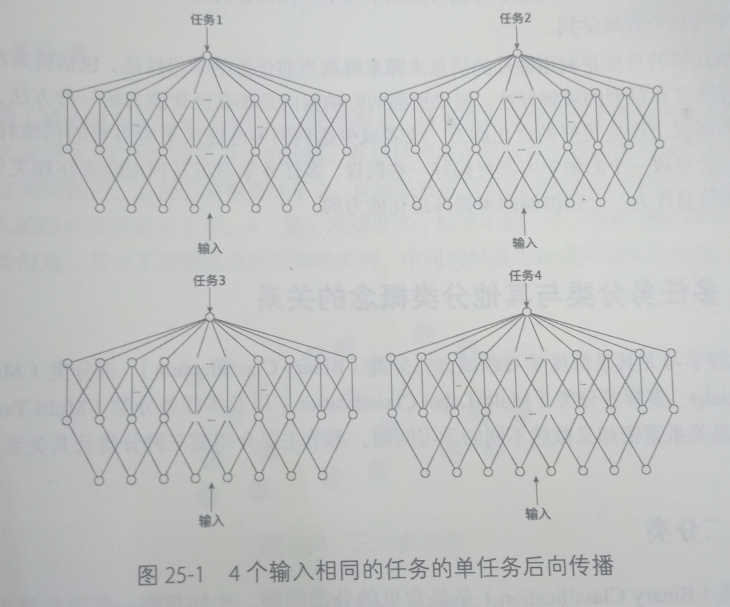下图展示了以恶搞输入于上图中的4个网络一致的单一网络，但该网络由4个输出，每个输出对应于上图的一个任务。

需要注意的是，这些输出可以连接他们共享的一个隐层的所有神经单元，也由如上图所示，在共享的一个隐层后形成一个独立的子网络，训练不与其他任务共享的参数。这里成为多任务学习。在多任务学习网络中，后向传播并行的作用于4个输出。由于4个输出共享底部的隐层，这些隐层中用于某个任务的特征表示也可以被其他任务利用，促使利用，促使多个任务共同学习。

多个任务并行训练共享不同任务以学习到的特征表示，是多任务学习的核心思想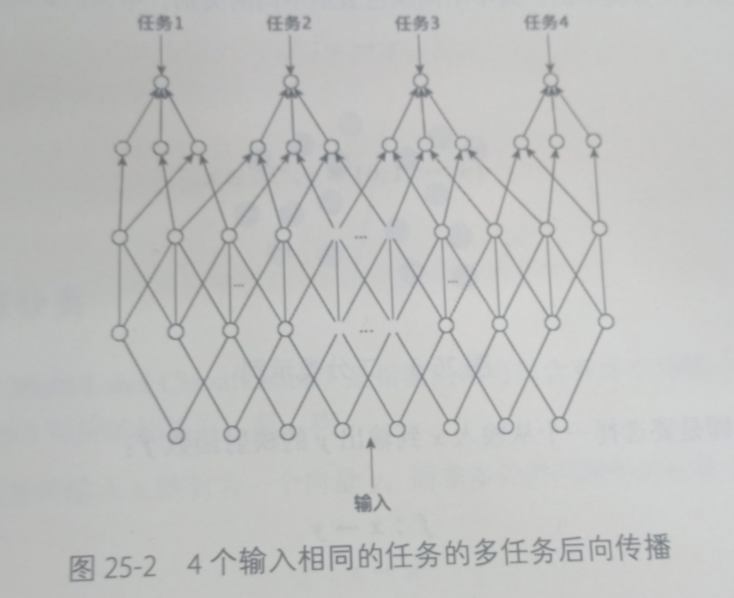### 二分类

对于二分类是常见的分类问题，比如判断一张图片是不是人脸。如下图所示是一个二分类示例，其中不同的颜色表示不同的类别，中间线对应的线性分类面。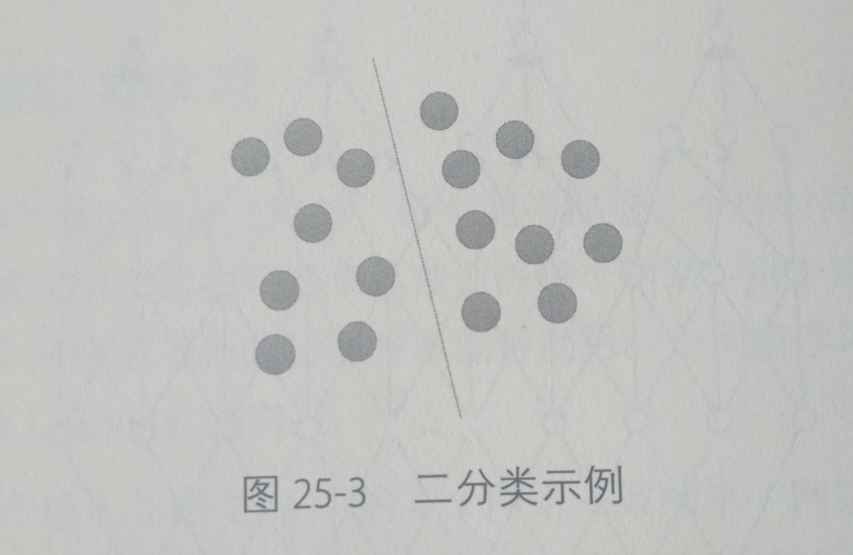### 多分类

多分类，是指分类数量大于2的分类问题。例如中文或者英文的OCR问题；在目标检测中针对每个候选框的分类是多分类问题。在这类问题中，总的标签数大于2，且每条数据只对应其中一个标签。比如识别问题，输入的图片必须对应于人、车、毛、狗、未知等多个标签的一个。如下图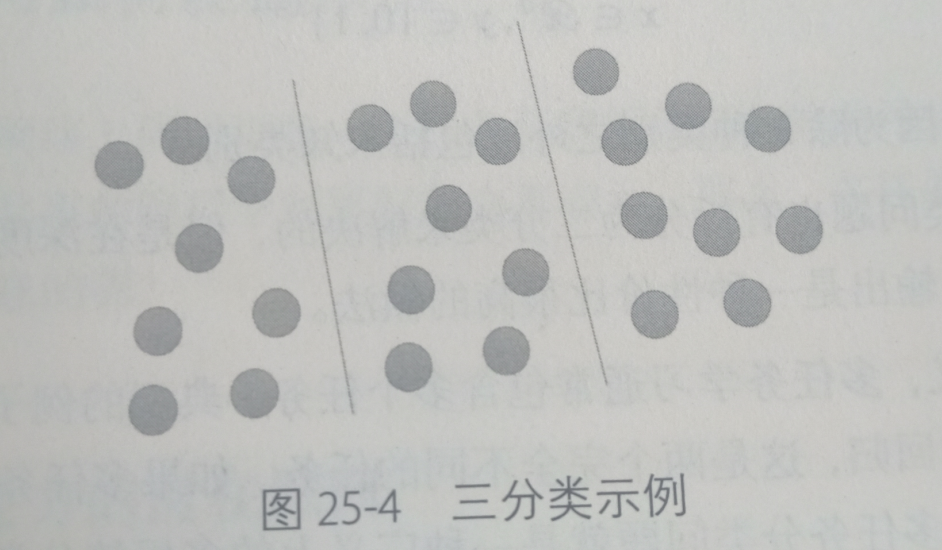### 多标签分类

针对多标签分类是指输入源可以含有多个物体，标注的标签也是有多个如下图传统的多标签分类问题也有拆分位二分类来解决的，但是在深度学习的今天，全连接后套多个Logistic（数据流）输出是一种性价比很高的做法。

多任务学习通常包含多个任务。最为典型的是Fast RCNN 同时做softmax分类和bbox的回归，这是两个完全不同的任务。如果多任务学习中的每个任务都为二分类问题，那么这种多分类问题就是一种广义上的多标签分类问题。

一般来说，多任务学习中不同的任务之间的区别较大，所以往往不共享所有层，比如 Fast RCNN 就是独立的两个全连接层，根据任务之间的区别大小，可以决定在不同的层开始分道扬镳。这种底层共享，高层分开的做法就是迁移学习。

### 相关关系

二分类、多分类、多标签分类、多任务分类、多任务学习、迁移学习的相互关系：

• 二分类是N= 2时的多分类
• 多分类时多标签分类的一种，时对多个相互独立的标签经行学习。
• 多标签时多任务学习的一种，每个任务对应一个标签。
• 多任务学习时迁移学习的一种，迁移学习中的源领域和目标领域对应多任务学习学习中的不同任务。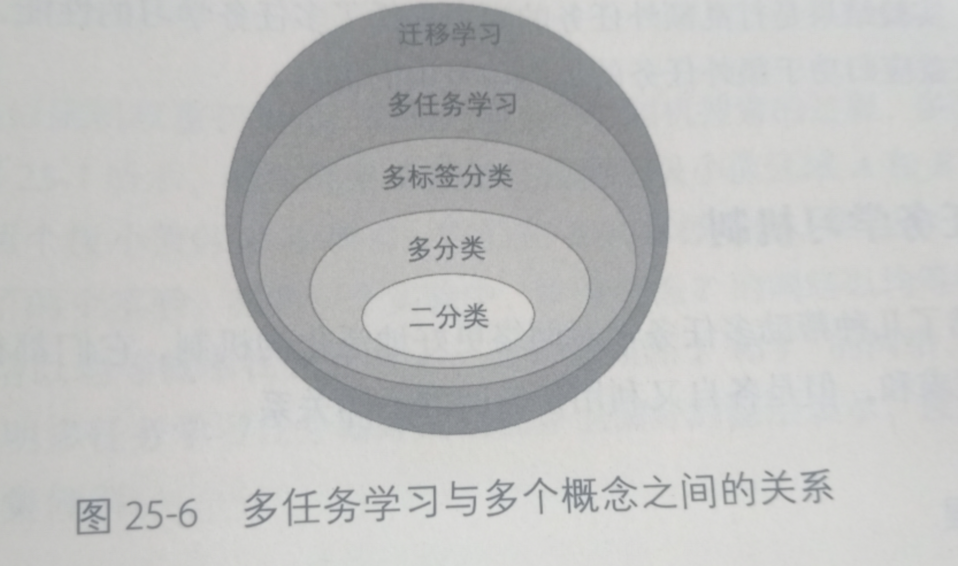希望这篇文章让你对深度学习或者机器学习更加深入。
谢谢点赞评论！

展开全文语音识别 深度学习 神经网络 多任务学习
• 单标签二分类算法，单标签多分类算法，多标签多分类算法及多标签多分类在Scikit-learn中的实现方式。

## 1、单标签二分类算法

单标签二分类这种问题是我们最常见的算法问题，主要是指label标签的取值只有两种，并且算法中只有一个需要预测的label标签。直白来讲就是每个实例的可能类别只有两种（A or B）。此时的分类算法其实是在构建一个分类线将数据划分为两个类别。常见的算法有：Logistic、SVM、KNN等。
y = f ( x ) ,       y ∈ { − 1 , + 1 } y=f(x),\ \ \ \ \ y\in\{-1, +1\}

###### Logistic算法

s i g m o i d = h θ ( x ) = 1 1 + e − θ T x J ( θ ) = − 1 m [ ∑ i = 1 m y ( i ) log ⁡ h θ ( x ( i ) ) + ( 1 − y ( i ) ) log ⁡ ( 1 − h θ ( x ( i ) ) ) ] ,       y ( i ) ∈ { 0 , 1 } \begin{aligned} sigmoid&=h_{\theta}(x) \\ &=\frac{1}{1+e^{-\theta^Tx}} \\ J(\theta)&=-\frac{1}{m}[\sum_{i=1}^my^{(i)}\log h_{\theta}(x^{(i)})+(1-y^{(i)})\log(1-h_{\theta}(x^{(i)}))], \ \ \ \ \ y^{(i)}\in\{0, 1\} \end{aligned}## 2、单标签多分类算法

单标签多分类问题其实是指待预测的label标签只有一个，但是label标签的取值可能有多种情况。直白来讲就是每个实例的可能类别有K种 ( t 1 , t 2 , … , t k , k ≥ 3 ) (t_1, t_2, \dots, t_k ,k\ge3) 。常见算法：Softmax、KNN等。
y = f ( x ) ,        y ∈ { t 1 , t 2 , … , t k } D = { ( x 1 , y 1 ) , ( x 2 , y 2 ) , … , ( x n , y n ) } y i = j ,      i = 1 , 2 , … , n      j = 1 , 2 , … , k \begin{aligned} y&=f(x),\ \ \ \ \ \ y\in\{t_1, t_2, \dots, t_k\} \\ D&=\{(x_1, y_1), (x_2, y_2), \dots, (x_n, y_n)\} \\ y_i&=j, \ \ \ \ i=1, 2, \dots, n \ \ \ \ j=1, 2, \dots, k \end{aligned}

###### Softmax算法

p ( y = k ∣ x ; θ ) = e θ k T x ∑ l = 1 K e θ l T x ,       k = 1 , 2 , … , K h θ ( x ) = [ p ( y ( i ) = 1 ∣ x ( i ) ; θ ) p ( y ( i ) = 2 ∣ x ( i ) ; θ ) … p ( y ( i ) = k ∣ x ( i ) ; θ ) ] = 1 ∑ j = 1 k e θ j T x ( i ) ⋅ [ e θ 1 T x e θ 2 T x … e θ k T x ] ⟹ θ = [ θ 11 θ 12 ⋯ θ 1 n θ 21 θ 22 ⋯ θ 2 n ⋯ ⋯ ⋯ ⋯ θ k 1 θ k 2 ⋯ θ k n ] J ( θ ) = − 1 m ∑ i = 1 m ∑ j = 1 k I ( y ( i ) = j ) log ⁡ ( e θ j T x ( i ) ∑ l = 1 K e θ l T x ) I ( y ( i ) = j ) = { 1 , y ( i ) = j 0 , y ( i ) ≠ j \begin{aligned} p(y=k|x;\theta)&=\frac{e^{\theta_k^Tx}}{\sum_{l=1}^Ke^{\theta_l^Tx}}, \ \ \ \ \ k=1, 2, \dots, K \\ h_{\theta}(x)&=\begin{bmatrix} p(y^{(i)}=1|x^{(i)};\theta) \\ p(y^{(i)}=2|x^{(i)};\theta) \\ \dots \\ p(y^{(i)}=k|x^{(i)};\theta) \end{bmatrix} \\ &=\frac{1}{\sum_{j=1}^ke^{\theta_j^Tx^{(i)}}}\cdot\begin{bmatrix} e^{\theta_1^Tx} \\ e^{\theta_2^Tx} \\ \dots \\ e^{\theta_k^Tx} \end{bmatrix} \\ \Longrightarrow \theta&=\begin{bmatrix} \theta_{11} & \theta_{12} & \cdots & \theta_{1n} \\ \theta_{21} & \theta_{22} & \cdots & \theta_{2n} \\ \cdots & \cdots & \cdots & \cdots \\ \theta_{k1} & \theta_{k2} & \cdots & \theta_{kn} \end{bmatrix} \\ J(\theta)&=-\frac{1}{m}\sum_{i=1}^m\sum_{j=1}^kI(y^{(i)}=j)\log(\frac{e^{\theta_j^Tx^{(i)}}}{\sum_{l=1}^Ke^{\theta_l^Tx}}) \\ I(y^{(i)}=j)&=\begin{cases} 1, &y^{(i)}=j \\ 0, &y^{(i)}\ne j \end{cases} \end{aligned}
在实际的工作中，如果是一个多分类的问题，我们可以将这个待求解的问题转换为二分类算法的延伸，即将多分类任务拆分为若干个二分类任务求解，具体的策略如下：
（1）One-Versus-One（ovo）：一对一
将K个类别中的两两类别数据进行组合，然后使用组合后的数据训练出来一个模型，从而产生K(K-1)/2个分类器，将这些分类器的结果进行融合，并将分类器的预测结果使用多数投票的方式输出最终的预测结果值。（2）One-Versus-All / One-Versus-the-Rest（ova/ovr）： 一对多
在一对多模型训练中，不是两两类别的组合，而是将每一个类别作为正例，其它剩余的样例作为反例分别来训练K个模型。然后在预测的时候，如果在这K个模型中，只有一个模型输出为正例，那么最终的预测结果就是属于该分类器的这个类别。如果产生多个正例，那么则可以选择根据分类器的置信度作为指标，来选择置信度最大的分类器作为最终结果，常见置信度：精确度、召回率。（3）Error Correcting Output codes（纠错码机制）：多对多
将模型构建应用分为两个阶段：编码阶段和解码阶段。编码阶段中对K个类别中进行M次划分，每次划分将一部分数据分为正类，一部分数据分为反类，每次划分都构建出来一个模型，模型的结果是在空间中对于每个类别都定义了一个点。解码阶段中使用训练出来的模型对测试样例进行预测，将预测样本对应的点和类别之间的点求距离，选择距离最近的类别作为最终的预测类别。## 3、多标签多分类算法

Multi-Label Machine Learning（MLL算法）是指预测模型中存在多个y值，具体分为两类不同情况：

• 多个待预测的y值
• 在分类模型中，一个样例可能存在多个不固定的类别

根据多标签业务问题的复杂性，可以将问题分为两大类：

• 待预测值之间存在相互的依赖关系
• 待预测值之间是不存在依赖关系的

对于这类问题的解决方案可以分为两大类：

• 转换策略（Problem Transformation Methods）

（1）Problem Transformation Methods
该方法又叫做策略转换或者问题转换，是一种将多标签的分类问题转换成为单标签模型构造的问题，然后将模型合并的一种方法，主要有以下几种方式：

• Binary Relevance（first-order）
• Classifier Chains（high-order）
• Calibrated Label Ranking（second-order）
###### Binary Relevance

Binary Relevance的核心思想是将多标签分类问题进行分解，将其转换为q个二元分类问题，其中每个二元分类器对应一个待预测的标签。
Binary Relevance方式的优点如下：

• 实现方式简单，容易理解
• 当y值之间不存在相关的依赖关系的时候，模型的效果不错

Binary Relevance方式的缺点如下：

• 如果y之间存在相互的依赖关系，那么最终构建的模型的泛化能力比较弱
• 需要构建q个二分类器，q为待预测的y值数量，当q比较大的时候，需要构建的模型会比较多
###### Classifier Chains

Classifier Chains的核心思想是将多标签分类问题进行分解，将其转换成为一个二元分类器链的形式，其中后链的二元分类器的构建是在前面分类器预测结果的基础上的。在模型构建的时候，首先将标签顺序进行shuffle打乱排序操作，然后按照从头到尾分别构建每个标签对应的模型。
Classifier Chains方法的优点如下：

• 实现方式相对比较简单，容易理解
• 考虑标签之间的依赖关系，最终模型的泛化能力相对于Binary Relevance方式构建的模型效果要好

缺点如下：

• 很难找到一个比较适合的标签依赖关系
###### Calibrated Label Ranking

Calibrated Label Ranking的核心思想是将多标签分类问题进行分解，将其转换为标签的排序问题，最终的标签就是排序后最大的几个标签值。
Calibrated Label Ranking 方法的优点如下：

• 考虑了标签两两组合的情况，最终的模型相对来讲泛化能力比较好

Calibrated Label Ranking 方法的缺点如下：

• 只考虑两两标签的组合，没有考虑到标签与标签之间的所有依赖关系

• ML-kNN
• ML-DT
###### ML-kNN

ML-kNN的思想：对于每一个实例来讲，先获取距离它最近的k个实例，然后使用这些实例的标签集合，通过最大后验概率（MAP）来判断这个实例的预测标签集合的值。
最大后验概率（MAP）：其实就是在最大似然估计（MLE）中加入了这个要估计量的先验概率分布。
θ ^ M L E ( x ) = arg ⁡ max ⁡ θ f ( x ∣ θ ) θ ^ M A P ( x ) = arg ⁡ max ⁡ θ f ( x ∣ θ ) g ( θ ) ∫ θ f ( x ∣ θ ′ ) g ( θ ′ ) d θ ′ = arg ⁡ max ⁡ θ f ( x ∣ θ ) g ( θ ) \begin{aligned} \hat{\theta}_{MLE}(x)&=\arg\max_{\theta}f(x|\theta) \\ \hat{\theta}_{MAP}(x)&=\arg\max_{\theta}\frac{f(x|\theta)g(\theta)}{\int_{\theta}f(x|\theta')g(\theta')d\theta'} \\ &=\arg\max_{\theta}f(x|\theta)g(\theta) \end{aligned}

###### ML-DT

ML-DT是使用决策树处理多标签内容，核心在于给予更细粒度的信息熵增益准则来构建这个决策树模型。
e n t r y = ∑ j = 1 q [ − p j log ⁡ p j − ( 1 − p j ) log ⁡ ( 1 − p j ) ] p j = ∑ i = 1 n ∣ ∣ y j ∈ Y i ∣ ∣ n \begin{aligned} entry&=\sum_{j=1}^q[-p_j\log p_j-(1-p_j)\log(1-p_j)] \\ p_j&=\frac{\sum_{i=1}^n||y_j\in Y_i||}{n} \end{aligned}

## 4、多标签多分类在Scikit-learn中的实现方式

在Scikit-learn中使用OneVsRestClassifier对多标签进行分类操作，内部其实是将多标签问题转换为多类别的区分问题。

展开全文• 详解sigmoid与softmax， 多分类及多标签分类激活函数介绍sigmoid激活函数sigmoid激活函数的性质sigmoid激活函数的使用 激活函数介绍 对于熟悉机器学习或神经网络的读者来说，sigmoid与softmax两个激活函数并不陌生，...

# 激活函数介绍

对于熟悉机器学习或神经网络的读者来说，sigmoid与softmax两个激活函数并不陌生，但这两个激活函数在逻辑回归中应用，也是面试和笔试会问到的一些内容，掌握好这两个激活函数及其衍生的能力是很基础且重要的，下面为大家介绍下这两类激活函数。

## sigmoid激活函数

从函数定义上来看，sigmoid激活函数的定义域能够取任何范围的实数，而返回的输出值在0到1的范围内。sigmoid函数也被称为S型函数，这是由于其函数曲线类似于S型，在下面的内容中可以看到。此外，该函数曲线也可以用于统计中，使用的是累积分布函数。

### sigmoid激活函数的性质

根据定义，sigmoid激活函数的计算公式如下：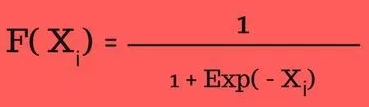其中:

• x: 输入
• float：表示浮点型数据
• exp：对其求指数
• f(x): 函数输出

从上述函数可以看到，x的取值范围可以是全实数，sigmoid函数返回一个实数值输出，此外，sigmoid函数的一阶导数是非负或非正：

• 非负： 如果输入数字大于或等于零；
• 非正： 如果输入数字小于或等于零；

### sigmoid激活函数的使用

• Sigmoid函数用于逻辑回归模型中的二进制分类。
• 在创建人造神经元时，Sigmoid函数用作激活函数。
• 在统计学中，S形函数图像是常见的累积分布函数。

### sigmoid激活函数python实现并画图

#### 实现代码

# Required Python Package
import numpy as np

def sigmoid(inputs):
"""
Calculate the sigmoid for the give inputs (array)
:param inputs:
:return:
"""
sigmoid_scores = [1 / float(1 + np.exp(- x)) for x in inputs]
return sigmoid_scores

sigmoid_inputs = [2, 3, 5, 6]
print "Sigmoid Function Output :: {}".format(sigmoid(sigmoid_inputs))


上是Sigmoid函数的实现代码。该函数将以列表形式的值作为输入参数。列表中的每个元素值将被视为Sigmoid函数的输入，并计算输出值。
接下来，我们将一个列表sigmiod_inputs作为函数的输入，列表值为2,3,5,6，经过sigmoid函数计算后获得Sigmoid分数。
函数输出：

Sigmoid Function Output :: [0.8807970779778823, 0.9525741268224334, 0.9933071490757153, 0.9975273768433653]


#### 画图

现在使用上面的函数来创建图像，以方便了解Sigmoid函数的性质。传递一个包含0到21范围内的数字的列表，计算输入列表的sigmoid分数，然后使用输出值来显示图像。

# Required Python Packages
import numpy as np
import matplotlib.pyplot as plt

def sigmoid(inputs):
"""
Calculate the sigmoid for the give inputs (array)
:param inputs:
:return:
"""
sigmoid_scores = [1 / float(1 + np.exp(- x)) for x in inputs]
return sigmoid_scores

def line_graph(x, y, x_title, y_title):
"""
Draw line graph with x and y values
:param x:
:param y:
:param x_title:
:param y_title:
:return:
"""
plt.plot(x, y)
plt.xlabel(x_title)
plt.ylabel(y_title)
plt.show()

graph_x = range(0, 21)
graph_y = sigmoid(graph_x)

line_graph(graph_x, graph_y, "Inputs", "Sigmoid Scores")


创建一个包含0到21范围内的数字的graph_x列表，之后在graph_y列表中存储给定graph_x输入的计算sigmoid分数，调用line_graph函数，该函数采用图像的x，y和标题来创建线形图。
脚本输出：

Graph X readings: [0, 1, 2, 3, 4, 5, 6, 7, 8, 9, 10, 11, 12, 13, 14, 15, 16, 17, 18, 19, 20]

Graph Y readings: [0.5, 0.7310585786300049, 0.8807970779778823, 0.9525741268224334, 0.9820137900379085, 0.9933071490757153, 0.9975273768433653, 0.9990889488055994, 0.9996646498695336, 0.9998766054240137, 0.9999546021312976, 0.999983298578152, 0.9999938558253978, 0.999997739675702, 0.9999991684719722, 0.999999694097773, 0.9999998874648379, 0.9999999586006244, 0.9999999847700205, 0.9999999943972036, 0.9999999979388463]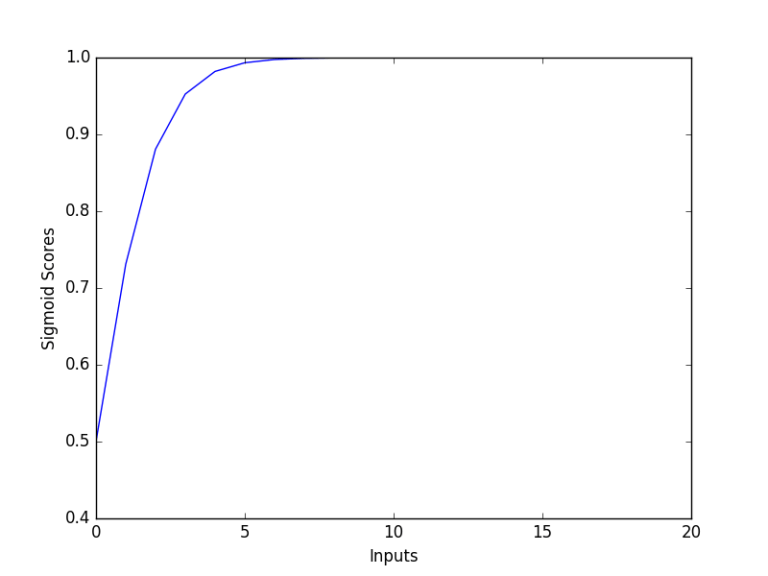从上图可以看出，随着输入值的增加，sigmoid得分增加到1。

## softmax激活函数

Softmax函数计算事件超过’n’个不同事件的概率分布。一般来说，这个函数将会计算每个目标类别在所有可能的目标类中的概率。计算出的概率将有助于确定给定输入的目标类别。
使用Softmax的主要优点是输出概率的范围，范围为0到1，所有概率的和将等于1。如果将softmax函数用于多分类模型，它会返回每个类别的概率，并且目标类别的概率值会很大。指数公式计算给定输入值的指数和输入中所有值的指数值之和。那么输入值的指数与指数值之和的比值就是softmax函数的输出。

### softmax激活函数的性质

根据定义，softmax激活函数的计算公式如下：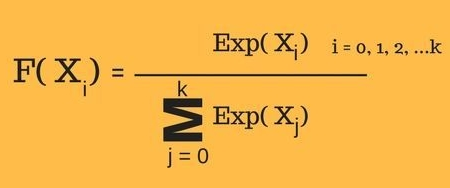其中:

• x: 输入
• exp：对其求指数
• f(x): 函数输出
从上述计算公式可以看出：
• 计算出的概率将在0到1的范围内。
• 所有概率的和等于1。

### softmax激活函数的使用

• 用于多重分类逻辑回归模型。
• 在构建神经网络中，在不同的层使用softmax函数。

### softmax激活函数python实现并画图

#### 实现代码

# Required Python Package
import numpy as np

def softmax(inputs):
"""
Calculate the softmax for the give inputs (array)
:param inputs:
:return:
"""
return np.exp(inputs) / float(sum(np.exp(inputs)))

softmax_inputs = [2, 3, 5, 6]
print "Softmax Function Output :: {}".format(softmax(softmax_inputs))


脚本输出:

Softmax Function Output :: [ 0.01275478  0.03467109  0.25618664  0.69638749]


从中可以观察到，输入值为6时，函数输出值的概率最高，这是可以从softmax函数预先知道的。之后在分类任务中，可以使用高概率值来预测给定输入特征的目标类别。

#### 画图

现在让我们使用实现的Softmax函数创建图像来了解这个函数的表现。
创建一个包含0到21范围内的值的列表，之后将通过此列表来计算已实现函数的分数，使用列表和估计分数创建图像。

# Required Python Packages
import numpy as np
import matplotlib.pyplot as plt

def softmax(inputs):
"""
Calculate the softmax for the give inputs (array)
:param inputs:
:return:
"""
return np.exp(inputs) / float(sum(np.exp(inputs)))

def line_graph(x, y, x_title, y_title):
"""
Draw line graph with x and y values
:param x:
:param y:
:param x_title:
:param y_title:
:return:
"""
plt.plot(x, y)
plt.xlabel(x_title)
plt.ylabel(y_title)
plt.show()

graph_x = range(0, 21)
graph_y = softmax(graph_x)

line_graph(graph_x, graph_y, "Inputs", "Softmax Scores")


脚本输出：

Graph X readings: [0, 1, 2, 3, 4, 5, 6, 7, 8, 9, 10, 11, 12, 13, 14, 15, 16, 17, 18, 19, 20]
Graph Y readings: [ 1.30289758e-09 3.54164282e-09 9.62718331e-09 2.61693975e-08 7.11357976e-08 1.93367146e-07 5.25626399e-07 1.42880069e-06 3.88388295e-06 1.05574884e-05 2.86982290e-05 7.80098744e-05 2.12052824e-04 5.76419338e-04 1.56687021e-03 4.25919483e-03 1.15776919e-02 3.14714295e-02 8.55482149e-02 2.32544158e-01 6.32120559e-01]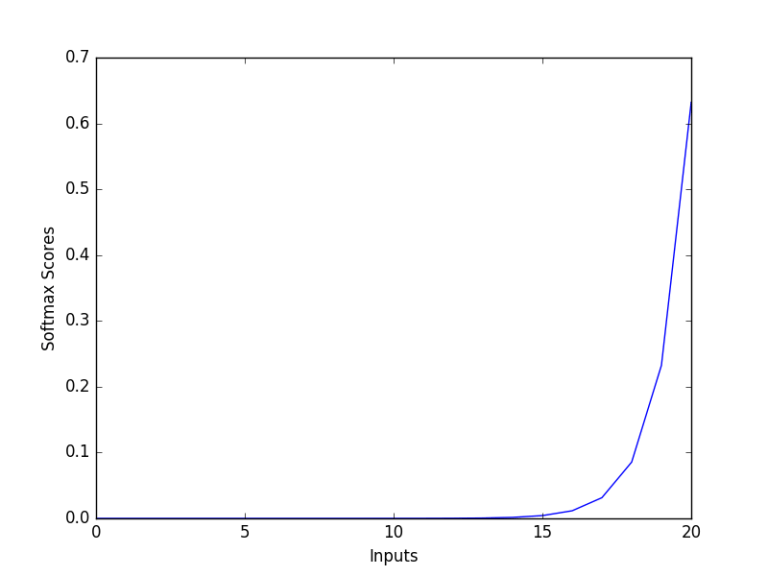该图显示了softmax函数的基本属性，输入值越大，其概率越高。

# 多类分类及多标签分类

多类分类意味着候选集是一个多分类，而不仅仅是二分类，不是是与否的问题，而是属于多类中哪一类的问题。一个样本属于且只属于多个分类中的一个，一个样本只能属于一个类，不同类之间是互斥的。举例而言，MNIST数据集，常用的数字手写体识别数据集，它的标签是一个多分类的过程，要将数字手写体识别为0~9中的某一个数字：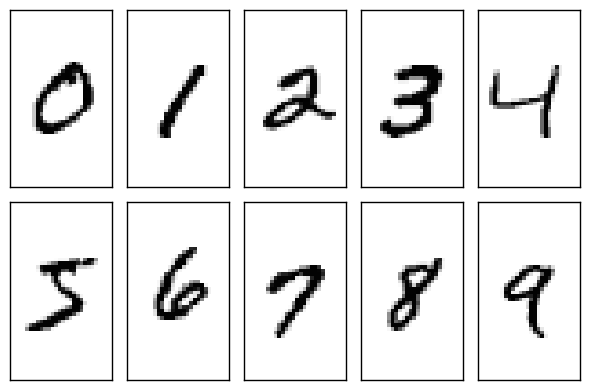而对于多标签分类而言，一个样本的标签不仅仅局限于一个类别，可以具有多个类别，不同类之间是有关联的。比如一件衣服，其具有的特征类别有长袖、蕾丝等属性等，这两个属性标签不是互斥的，而是有关联的。

# 使用softmax和sigmoid激活函数来做多类分类和多标签分类

在实际应用中，一般将softmax用于多类分类的使用之中，而将sigmoid用于多标签分类之中，对于图像处理而言，网络模型抽取图像特征的结构基本相同，只是根据不同的任务改变全连接层后的输出层。下面介绍如何使用softmax和sigmoid完成对应的分类任务。

## softmax激活函数应用于多类分类

假设神经网络模型的最后一层的全连接层输出的是一维向量logits=[1,2,3,4,5,6,7,8,9,10],这里假设总共类别数量为10，使用softmax分类器完成多类分类问题，并将损失函数设置为categorical_crossentropy损失函数：
用tensorflow实现：

tf.argmax(tf.softmax(logits))


首先用softmax将logits转换成一个概率分布，然后取概率值最大的作为样本的分类 。softmax的主要作用其实是在计算交叉熵上，将logits转换成一个概率分布后再来计算，然后取概率分布中最大的作为最终的分类结果，这就是将softmax激活函数应用于多分类中。

## sigmoid激活函数应用于多标签分类

sigmoid一般不用来做多类分类，而是用来做二分类，它是将一个标量数字转换到[0,1]之间，如果大于一个概率阈值(一般是0.5)，则认为属于某个类别，否则不属于某个类别。这一属性使得其适合应用于多标签分类之中，在多标签分类中，大多使用binary_crossentropy损失函数。它是将一个标量数字转换到[0,1]之间，如果大于一个概率阈值(一般是0.5)，则认为属于某个类别。本质上其实就是针对logits中每个分类计算的结果分别作用一个sigmoid分类器，分别判定样本是否属于某个类别同样假设，神经网络模型最后的输出是这样一个向量logits=[1,2,3,4,5,6,7,8,9,10], 就是神经网络最终的全连接的输出。这里假设总共有10个分类。通过：

tf.sigmoid(logits)


sigmoid应该会将logits中每个数字都变成[0,1]之间的概率值，假设结果为[0.01, 0.05, 0.4, 0.6, 0.3, 0.1, 0.5, 0.4, 0.06, 0.8], 然后设置一个概率阈值，比如0.3，如果概率值大于0.3，则判定类别符合，那么该输入样本则会被判定为类别3、类别4、类别5、类别7及类别8。即一个样本具有多个标签。
在这里强调一点：将sigmoid激活函数应用于多标签分类时，其损失函数应设置为binary_crossentropy。

展开全文softmax 多标签分类
• matlab实现的多分类逻辑回归，用于手写数字识别
• 将路透社新闻划分为 46 个互斥的主题，因为有多个类别，所以这是多分类（multiclass classification）问题的一个例子。因为每个数据点只能划分到一个类别，所以更具体地说，这是单标签、多分类（single-label, ...keras 深度学习
• 原理SVM被提出于1964年，在二十世纪90年代后得到快速发展并衍生出一系列改进和扩展算法，在人像识别、文本分类等模式识别(pattern recognition)问题中有得到应用。支持向量机(Support Vector Machine, SVM)是一类按...
• matlab 使用svm进行分类含实例代码（适用于二分类和多分类matlab 支持向量机 matlab svm svm
• 一直想写篇度量指标的文章，梳理一下这块的知识点，可能想了太多次，总以为自己已经写过了，今天看文章列表，竟然没有相关的内容，刚好最近在做多分类模型，借此机会整理一下。 混淆矩阵（confusion matrix） 在...机器学习
• 分类评价指标 准确率（Accuracy） 评价分类问题的性能指标一般是分类准确率，即对于给定的数据，分类正确的样本数占总样本数的比例。 注意：准确率这一指标在Unbalanced数据集上的表现很差，因为如果我们的正负...评价指标
• 多分类问题在最后的输出层采用的Softmax Layer，其具有两个特点：1.每个输出的值都是在(0,1)；2.所有值加起来和为1. 假设是最后线性层的输出，则对应的Softmax function为： 输出经过sigmoid运算即可是西安输出...pytorch 深度学习 人工智能
• 多分类： 一、什么是多类分类？ 二、如何处理多类分类？ 三、代码实践： 评估指标：混淆矩阵，accuracy，precision，f1-score，AUC，ROC，P-R（不能用） 1.混淆矩阵： 2. accuracy，precision，reacall，f1-score： ...sklearn 机器学习
• ## 基于LSTM的中文文本多分类实战

万次阅读 多人点赞 2019-05-06 00:30:42
在我之前的博客中我们介绍了文本的多分类的方法,我们还尝试了各种分类模型,比如朴素贝叶斯、逻辑回归、支持向量机和随机森林等并且都取得了非常不错的效果。今天我们使用深度学习中的LSTM（Long Short-Term Memory）...LSTM
• 理解关键点一 二分类和多分类的交叉熵的区别 二分类交叉熵 多分类交叉熵 理解关键点二 多分类和二分类focal loss 理解关键点三 alpha到底该如何设置focal loss 损失函数 深度学习
• 目录二分类逻辑回归数据准备模型构建模型检验多分类逻辑回归 二分类逻辑回归 首先，我先展示下我逻辑回归的总体代码，如果有基础的同志需要的话，可以直接修改数据和参数拿去用呀： library(lattice) library(ggplot...r语言 逻辑回归
• 2. 多分类评价指标 3. 总结 1. 二分类评价指标 常用的二分类评价指标包括准确率、精确率、召回率、F1-score、AUC、ROC、P-R曲线、MCC等 混淆矩阵 2分类问题的混淆矩阵是2*2的，通常以关注的类为正类，另一个类为...深度学习
• 逻辑回归知识要点一、逻辑回归模型模型简介：算法的分类思想算法模型sigmoid函数函数原型sigmoid函数图像参数求解二、逻辑回归实现二分类模型训练与预测结果可视化计算概率值绘制决策边界三、逻辑回归实现多分类建模...python 机器学习
• 本文主要记录一下pytorch里面的二分类及多分类交叉熵损失函数的使用。 import torch import torch.nn as nn import torch.nn.functional as F torch.manual_seed(2020) <torch._C.Generator at 0x7f4e8b3298b0&...pytorch
• 本文介绍了利用BP神经网络实现对不同半径的圆进行多分类（3分类），特征即为圆的半径。 输入层12节点，一个6节点的隐藏层，输出层3个节点。
• ## 多分类及多标签分类算法

万次阅读 多人点赞 2019-04-08 19:49:55
一、单标签多分类 1、单标签二分类算法原理 1、单标签二分类这种问题是我们最常见的算法问题，主要是指label 标签的取值只有两种，并且算法中只有一个需要预测的label标签； 直白来讲就是每个实例的可能类别...机器学习
• 对于组合二元支持向量机模型的类学习，使用纠错输出码（ECOC，error-correcting output codes ）。有关详细信息，请参阅fitcecoc。 ECOC 可以用来将 Multiclass Learning 问题转化为 Binary Classification 问题。...matlab SVM
• pred = [np.argmax(y) for y in Y_pred] # 取出y中元素最大值所对应的索引 Y_valid = [np.argmax(y) for y in Y_valid] # micro：多分类　# weighted：不均衡数量的类来说，计算二分类metrics的平均 # macro：计算二...python 二分类 ROC曲线 keras
• ## 多分类ROC曲线

万次阅读 多人点赞 2019-02-14 11:13:38
一个分类模型(分类器)是一个将某个实例映射到一个特定类的过程.分类器的结果可以是给出该实例所属的类别，也可以给定该实例属于某个类别的概率。 首先来考虑一个两类预测问题(双分类器),其结果要么是真(p)要么是假...
• 而针对多分类问题来说，有些二分类的评价准则就相对而言不怎么适用了。虽然可以将多分类问题转化为多个2vs2问题进行讨论，步骤繁杂的同时效果也得不到保障。目前在进行多模态的一个分类研究，在模型评价时也废了不少...
• 二、这个就是多分类中的交叉熵损失函数。 三、而二分类中的交叉熵损失一般写成这样：1 四、看起来二分类和多分类为什么不一样? 貌似不一样，二分类为什么多一项?主要是one-hot向量搞得鬼！ 如果按多分类中的one-...逻辑回归 深度学习 二分类
• ## Svm实现多分类

万次阅读 多人点赞 2019-05-14 17:17:23
Svm实现多分类Svm实现多类分类原理代码实现训练的图片 Svm实现多类分类原理 1.支持向量机分类算法最初只用于解决二分类问题，缺乏处理多分类问题的能力。后来随着需求的变化，需要svm处理多分类分为。目前构造多分类...
• ## 应用XGboost实现多分类模型实践

万次阅读 热门讨论 2019-04-04 16:44:45
本文把XGBoost集成算法模型，应用在工业生产中，分析生产过程数据，提高...实验学习目标采用XGBoost多分类multi:softprob输出概率，在8000个样本条件下，训练模型，达到62%准确率。本文分享了实验中关键点和填过的坑。机器学习 XGBoost Python 预测概率
• ## ROC曲线绘制(python+sklearn+多分类)

千次阅读 热门讨论 2020-07-29 13:06:51
目录 ROC曲线绘制要点（仅记录） 1、ROC用于度量模型性能 2、用于二分类问题，如若遇到多分类也以二分类的思想进行操作。 3、二分类问题代码实现（至于实现，文档说的很清楚了:[官方文档]...sklearn python
• 2. 多分类问题 3. 多标签分类问题 参考文章 1. 基本概念 2分类 分类任务有两个类别，每个样本属于两个类别中的一个，标签0/1.比如：训练一个图像分类器，判断一张输入图片是否是猫。 多分类 分类任务有n个...深度学习 多标签分类...